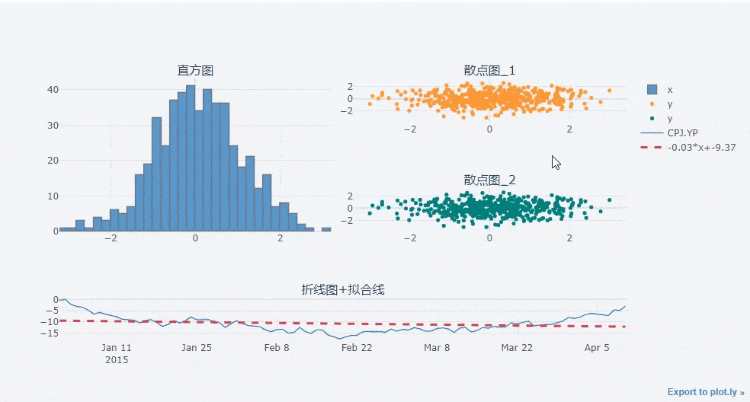# Tips: teach you to generate 30 cool dynamic interactive charts with one click of pandas

2022-02-02 02:51:08

Today, let's talk about how to use one line of code in `DataFrame` Generate cool dynamic interactive charts in the dataset , Let's first introduce the modules we need to use this time `cufflinks`
like `seaborn` Encapsulates the `matplotlib` equally ,`cufflinks` Also in the `plotly` Further packaging and optimization are made above , The methods are unified 、 Simple parameter configuration , about `DataFrame` It is also convenient and flexible for drawing data sets , And the chart we're going to draw this time includes

• Broken line diagram
• Area map
• Scatter plot
• Histogram
• Histogram
• Box figure
• Heat map
• 3D Scatter plot /3D Bubble chart
• Trend chart
• The pie chart
• K Line graph
• Multiple subgraphs are combined

### Module installation

Involving installation , direct `pip install` that will do

``````pip install cufflinks
Copy code ``````

### The import module , And view the relevant configuration

We import the module , Let's see what the current version is

``````cf.__version__
Copy code ``````

output

``````'0.17.3'
Copy code ``````

At present, the version of the module has reached `0.17.3`, It's also the latest version , Then, what charts can be drawn in our latest version

``````cf.help()
Copy code ``````

output

``````Use 'cufflinks.help(figure)' to see the list of available parameters for the given figure.
Use 'DataFrame.iplot(kind=figure)' to plot the respective figure
Figures:
bar
box
bubble
bubble3d
candle
choroplet
distplot
.......
Copy code ``````

From the output above we can see , The general syntax for drawing a chart is `df.iplot(kind= Chart name )` How do we want to view the parameters when drawing a specific chart , For example, a histogram `bar` What are the parameters , You can do that

``````cf.help('bar')
Copy code ``````

### Histogram

Let's first look at the drawing of histogram chart , First, let's create a data set for chart drawing

``````df2 = pd.DataFrame({'Category':['A','B','C','D'],
'Values':[95,56,70,85]})
df2
Copy code ``````

output

``````  Category  Values
0        A      95
1        B      56
2        C      70
3        D      85
Copy code ``````

Then let's draw the histogram

``````df2.iplot(kind='bar',x='Category',y='Values',
xTitle = "Category",yTitle = "Values",
title = " Histogram ")
Copy code ``````

output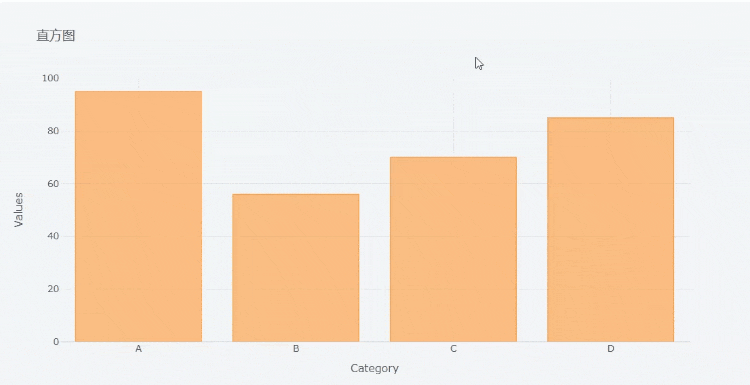Among them `x` The parameter is filled with `x` The corresponding variable name on the axis , and `y` The parameter is filled in `y` The corresponding variable name on the axis , We can draw the chart in `png` Download it in the format of ,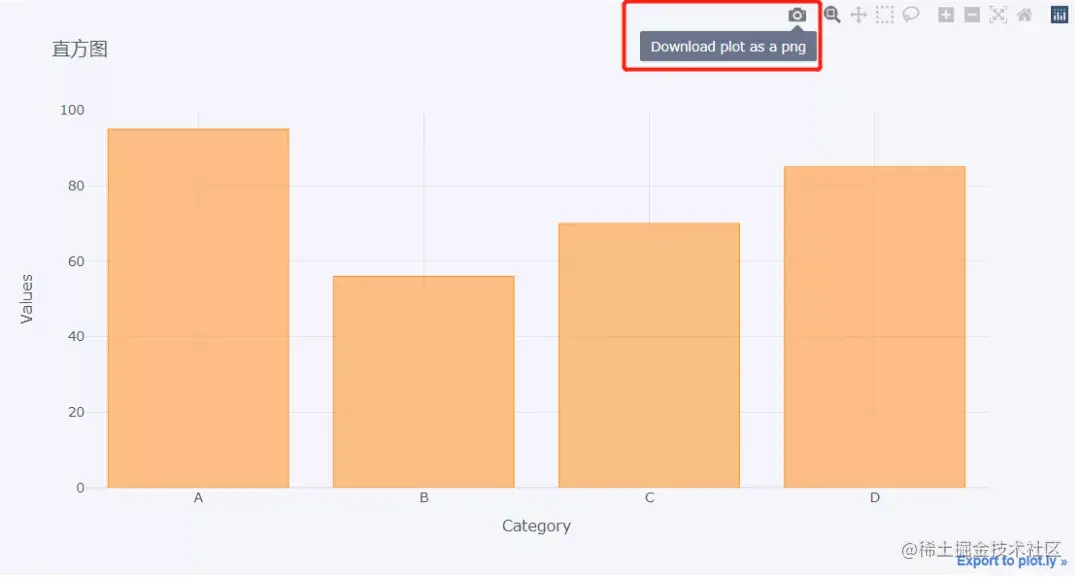At the same time, we can also zoom in on the chart ,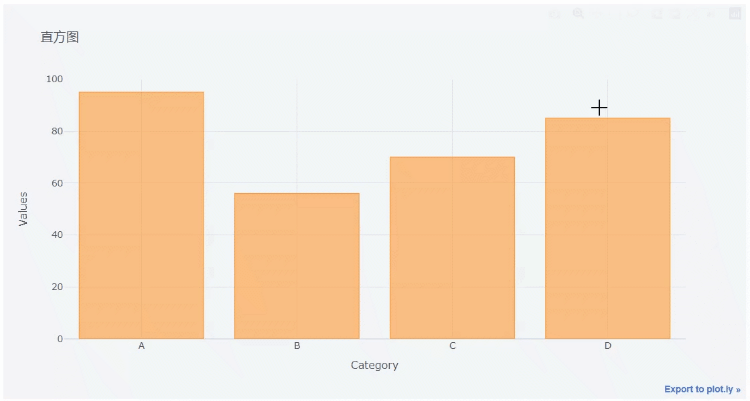Let's take a look at the following set of data

``````df = pd.DataFrame(np.random.randn(100,4),columns='A B C D'.split())
Copy code ``````

output

``````          A         B         C         D
0  0.612403 -0.029236 -0.595502  0.027722
1  1.167609  1.528045 -0.498168 -0.221060
2 -1.338883 -0.732692  0.935410  0.338740
3  1.662209  0.269750 -1.026117 -0.858472
4  1.387077 -0.839192 -0.562382 -0.989672
Copy code ``````

Let's plot the histogram

``````df.head(10).iplot('bar')
Copy code ``````

output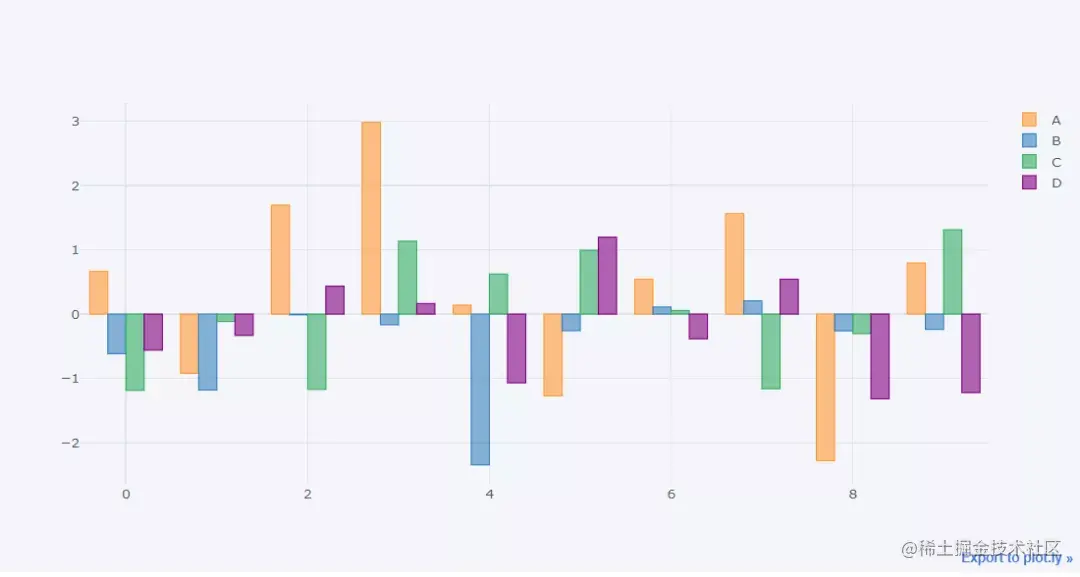We can also draw “ Stacked ” Histogram

``````df.head(10).iplot(kind='bar',barmode='stack')
Copy code ``````

output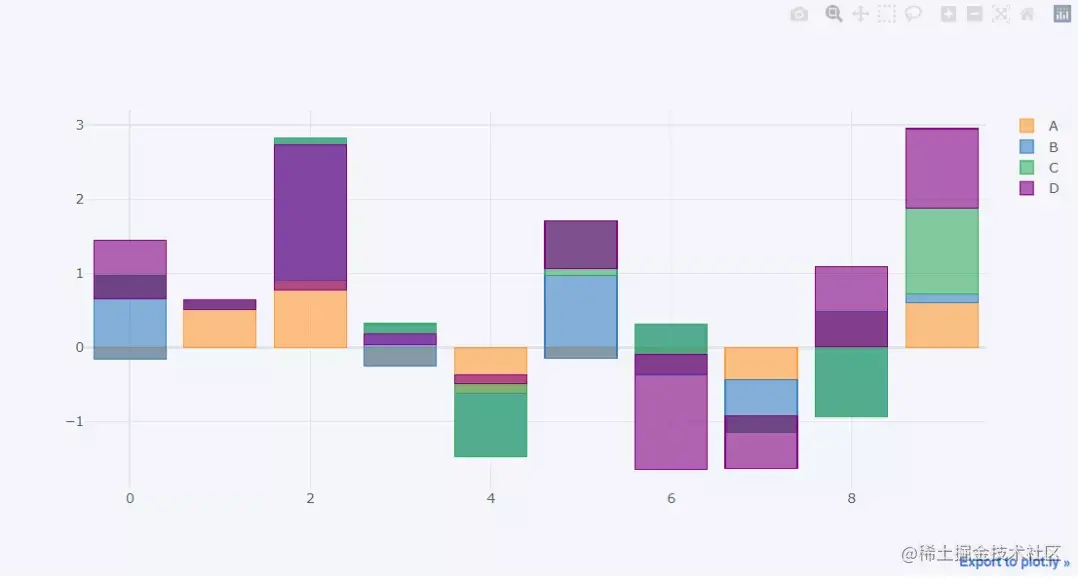So again , We can also draw the histogram horizontally

``````df.head(10).iplot(kind='barh',barmode='stack')
Copy code ``````

output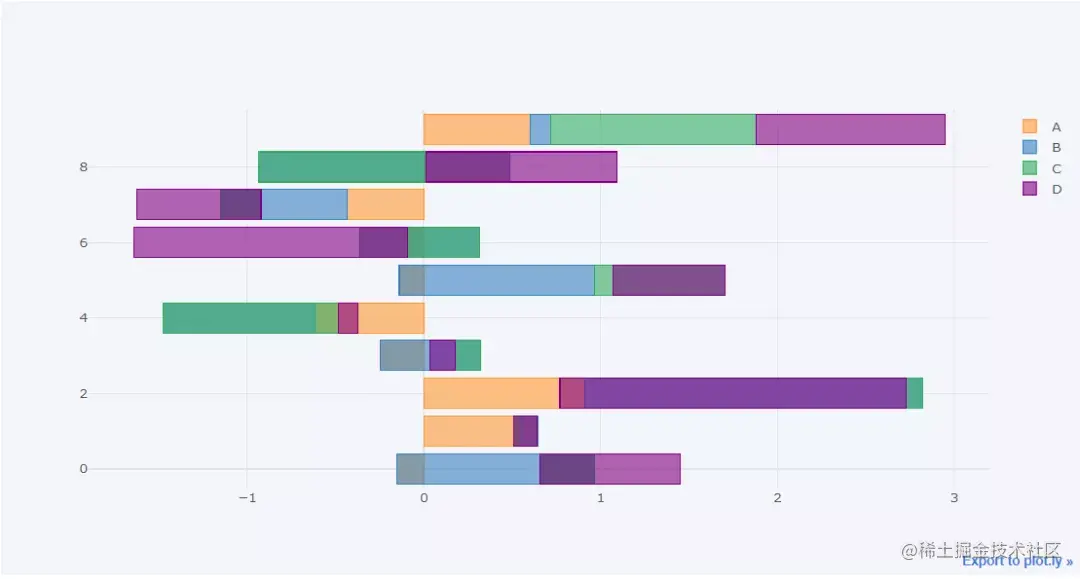### Broken line diagram

Now let's take a look at the drawing of line chart , Let's start with the above `df` The columns of the dataset are accumulated

``````df3 = df.cumsum()
Copy code ``````

Then let's draw a line chart

``````df3.iplot()
Copy code ``````

output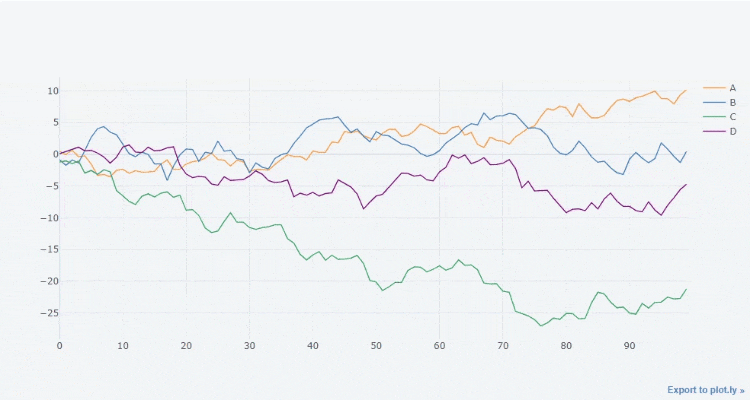Of course, you can also filter out a few columns and draw them , The effect is as follows

``````df3[["A", "B"]].iplot()
Copy code ``````

output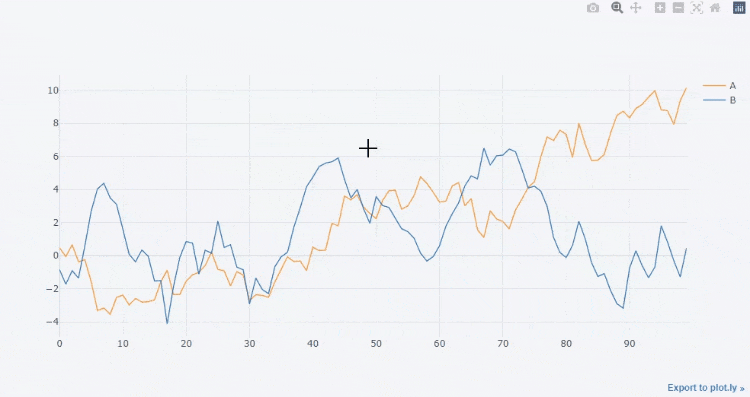We can also draw a straight line to fit its trend ,

``````df3['A'].iplot(bestfit = True,bestfit_colors=['pink'])
Copy code ``````

output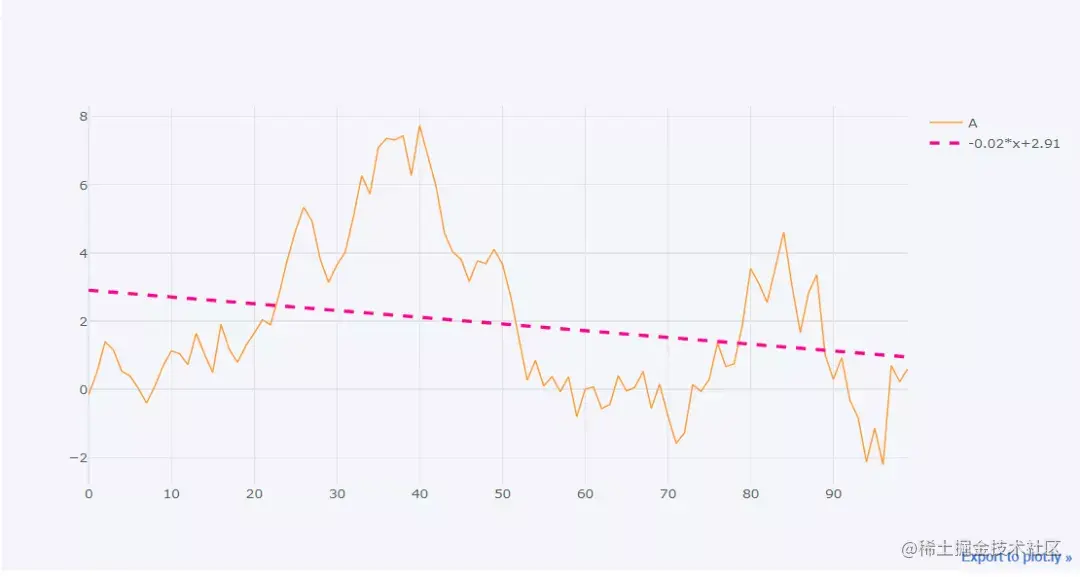Here we will focus on introducing a `iplot()` Parameters commonly used in methods

• `kind`: Chart type , The default is `scatter`, Scatter type , There are other types to choose from bar( Histogram )、box( Box figure )、heatmap( Heat map ) wait
• `theme`: Layout theme , Can pass `cf.getThemes()` To see what are the main
• `title`: The title of the chart
• `xTitle/yTitle`: x perhaps y The name of the shaft above the shaft
• `colors`: The color when drawing the chart
• `subplots`: Boolean value , When drawing subgraphs, you need , The default is `False`
• `mode`` character string `, Drawing mode , There can be `lines``markers`, There's also `lines+markers` and `lines+text` Equal mode
• `size`: For scatter charts , It is mainly used to adjust the size of scatter points
• `shape`: When drawing subgraphs, the layout of each graph
• `bargap`: The distance between columns in the histogram
• `barmode` : The shape of histogram ,stack( Stacked )、group( Clusters )、overlay( Cover )

### Area map

The transition from line graph to area graph is very simple , Only the parameters `fill` Set to `True` that will do , The code is as follows

``````df3.iplot(fill = True)
Copy code ``````

output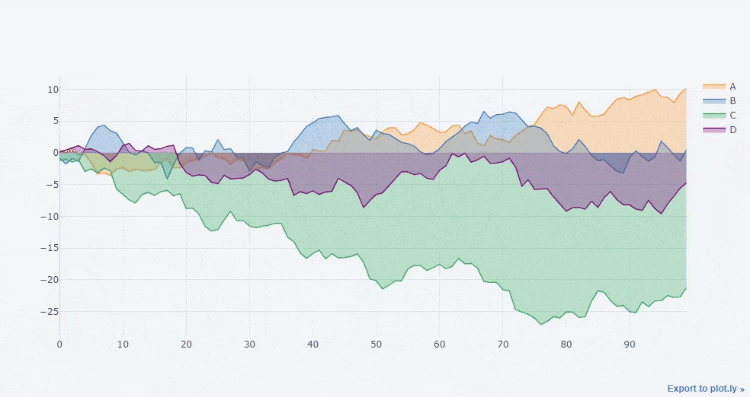### Scatter plot

For the drawing of scatter diagram , We need to `mode` Set to `marker`, The code is as follows

``````df3.iplot(kind='scatter',x='A',y='B',
mode='markers',size=10)
Copy code ``````

output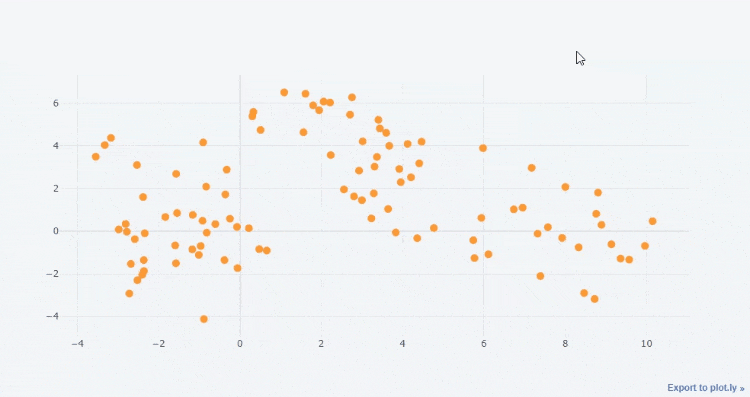We can adjust `size` Parameter to adjust the size of the scatter , For example, we will `size` Adjust to 20

``````df3.iplot(kind='scatter',x='A',y='B',
mode='markers',size=20)
Copy code ``````

output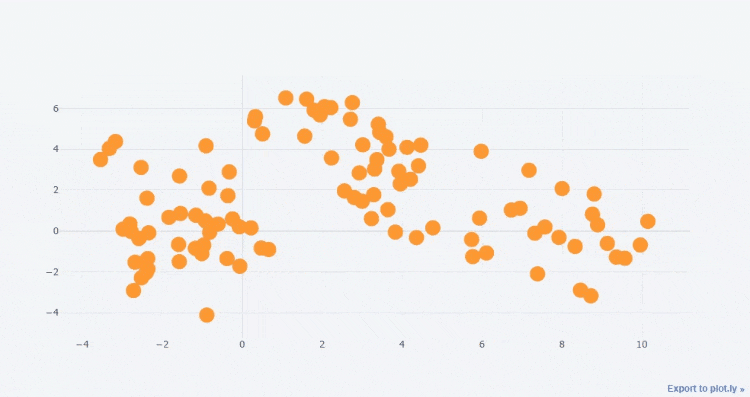Or will mode Set to `lines+markers`, The code is as follows

``````df3.iplot(kind='scatter',x='A',y='B',
mode='lines + markers',size=10)
Copy code ``````

We can also set the shape of the scatter , For example, the following code

``````df3.iplot(kind='scatter',x='A',y='B',
mode='markers',size=20,symbol="x",
colorscale='paired',)
Copy code ``````

output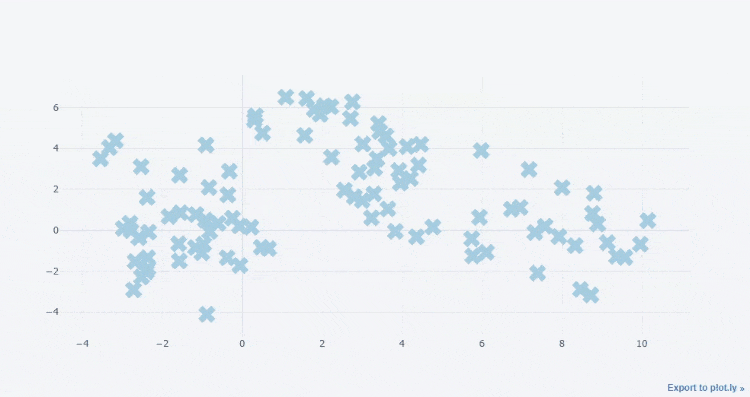Of course, we can also set the color of scatter

``````df.iplot(kind='scatter' ,mode='markers',
symbol='square',colors=['orange','purple','blue','red'],
size=20)
Copy code ``````

output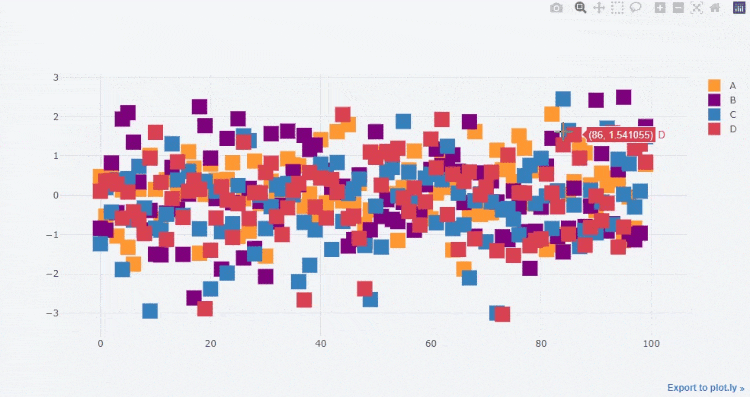### Bubble chart

The presentation of bubble chart is similar to that of scatter chart , On the drawing, you will `kind` Change the parameter to `bubble`, Suppose we have such a set of data

``````cf.datagen.bubble(prefix='industry').head()
Copy code ``````

output

``````          x         y  size    text categories
0  0.332274  1.053811     2  LCN.CG  industry1
1 -0.856835  0.422373    87  ZKY.XC  industry1
2 -0.818344 -0.167020    72  ZSJ.DJ  industry1
3 -0.720254  0.458264    11  ONG.SM  industry1
4 -0.004744  0.644006    40  HUW.DN  industry1
Copy code ``````

Let's draw a bubble chart

``````cf.datagen.bubble(prefix='industry').iplot(kind='bubble',x='x',y='y',size='size',
categories='categories',text='text', xTitle='Returns',
yTitle='Analyst Score',title='Cufflinks -  Bubble chart ')
Copy code ``````

output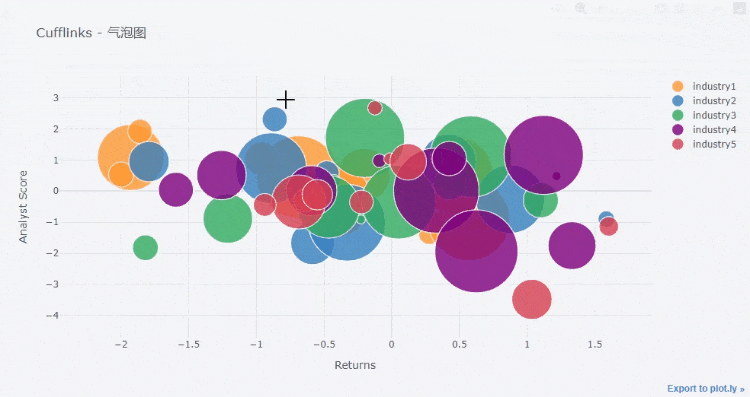The difference between bubble chart and scatter chart is , The size of each point in the scatter diagram is the same , But the bubble chart is not so

### 3D Scatter plot

Now that we've mentioned the bubble chart , that 3D By the way, the scatter chart , Suppose our data are as follows

``````cf.datagen.scatter3d(2,150).head()
Copy code ``````

output

``````          x         y         z    text categories
0  0.375359 -0.683845 -0.960599  RER.JD  category1
1  0.635806  1.210649  0.319687  INM.LE  category1
2  0.578831  0.103654  1.333646  BSZ.HS  category1
3 -1.128907 -1.189098  1.531494  GJZ.UX  category1
4  0.067668 -1.990996  0.088281  IQZ.KS  category1
Copy code ``````

Let's draw 3D Bubble chart of , Since it is a three-dimensional figure, it shows that there is x Axis 、y Axis and z Axis , The code is as follows

``````cf.datagen.scatter3d(2,150).iplot(kind='scatter3d',x='x',y='y',z='z',size=15,
categories='categories',text='text',
title='Cufflinks - 3D Bubble chart ',colors=['yellow','purple'],
width=1,margin=(0,0,0,0),
opacity=1)
Copy code ``````

output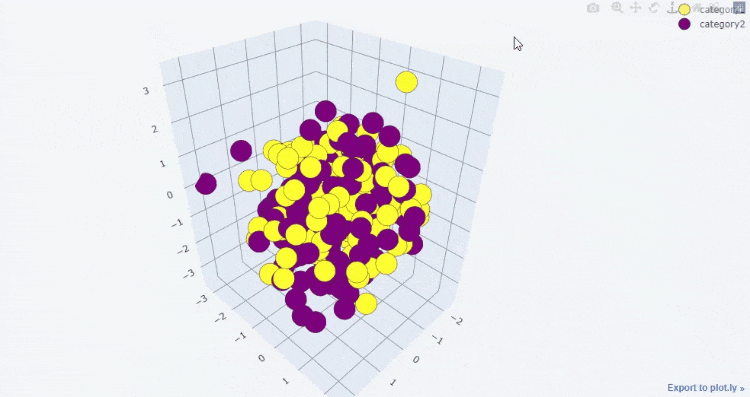### 3D Bubble chart

So it's mentioned that 3D Scatter plot , I have to say 3D Bubble chart of , Suppose our data set is this long

``````cf.datagen.bubble3d(5,4).head()
Copy code ``````

output

``````          x         y         z  size    text categories
0 -1.888528  0.801430 -0.493671    77  OKC.HL  category1
1 -0.744953 -0.004398 -1.249949    61  GAG.UH  category1
2  0.980846  1.241730 -0.741482    37  LVB.EM  category1
3 -0.230157  0.427072  0.007010    78  NWZ.MG  category1
4  0.025272 -0.424051 -0.602937    76  JDW.AX  category2
Copy code ``````

Let's draw 3D Bubble chart of

``````cf.datagen.bubble3d(5,4).iplot(kind='bubble3d',x='x',y='y',z='z',size='size',
text='text',categories='categories',
title='Cufflinks - 3D Bubble chart ',colorscale='set1',
width=.9,opacity=0.9)
Copy code ``````

output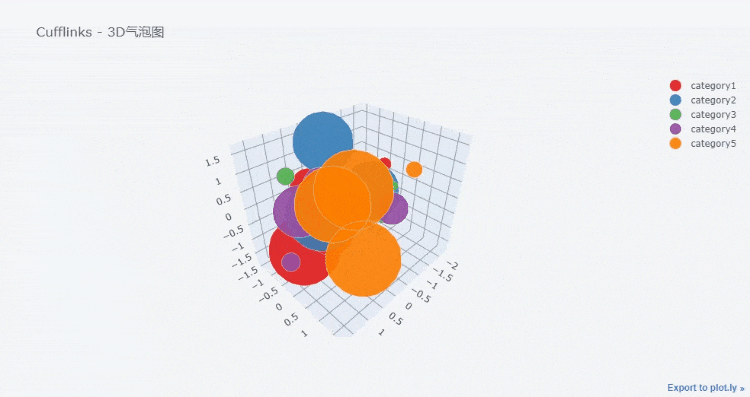### Box figure

Next, let's look at the drawing of the box diagram , The box chart is useful for us to observe the distribution of data 、 Whether there are extreme values is of great help

``````df.iplot(kind = "box")
Copy code ``````

output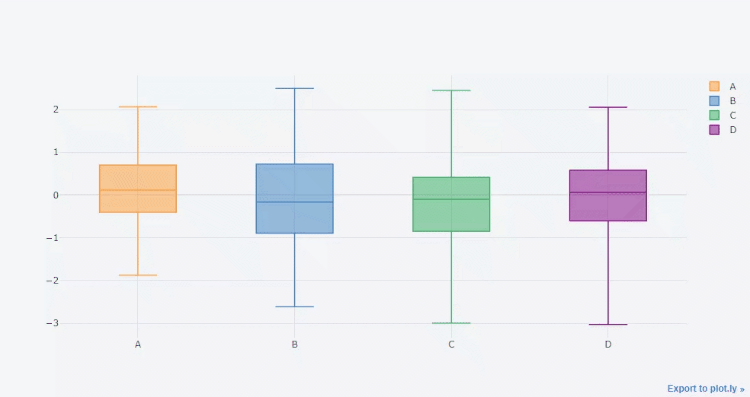### Heat map

This is the drawing of the heat map , Let's look at the dataset

``````cf.datagen.heatmap(20,20).head()
Copy code ``````

output

``````           y_0        y_1        y_2  ...       y_17       y_18       y_19
x_0  40.000000  58.195525  55.355233  ...  77.318287  80.187609  78.959951
x_1  37.111934  25.068114  25.730511  ...  27.261941  32.303315  28.550340
x_2  54.881357  54.254479  59.434281  ...  75.894161  74.051203  72.896999
x_3  41.337221  39.319033  37.916613  ...  15.885289  29.404226  26.278611
x_4  42.862472  36.365226  37.959368  ...  24.998608  25.096598  32.413760
Copy code ``````

Let's draw a heat map , The code is as follows

``````cf.datagen.heatmap(20,20).iplot(kind='heatmap',colorscale='spectral',title='Cufflinks -  Heat map ')
Copy code ``````

output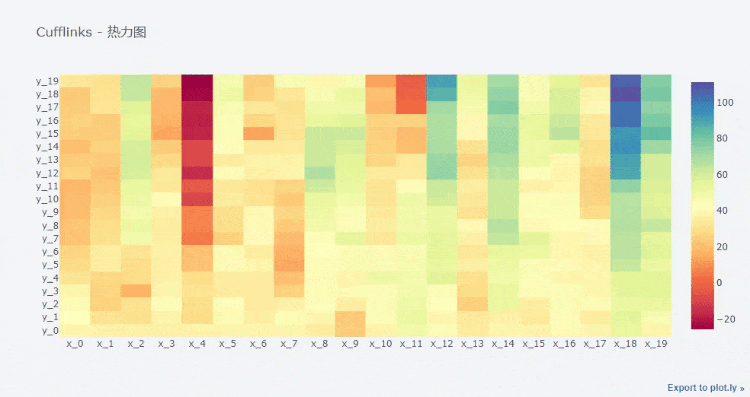### Trend chart

The so-called trend chart , To put it bluntly, it is the combination of line chart and area chart , The code is as follows

``````df[["A", "B"]].iplot(kind = 'spread')
Copy code ``````

output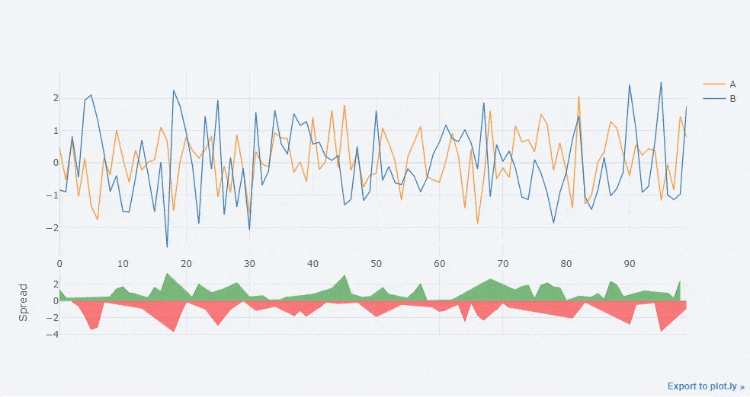### The pie chart

Now let's take a look at the drawing of pie chart , The code is as follows

``````cf.datagen.pie(n_labels=6, mode = "stocks").iplot(
kind = "pie",
labels = "labels",
values = "values")
Copy code ``````

output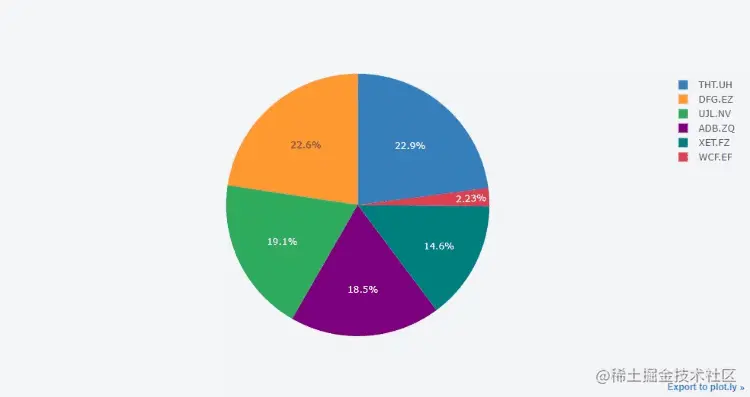### K Line graph

`cufflinks` It can also be used to draw K Line graph , Let's look at the data set here

``````cf.datagen.ohlc().head()
Copy code ``````

output

``````                  open        high         low       close
2015-01-01  100.000000  119.144561   97.305961  106.125985
2015-01-02  106.131897  118.814224   96.740816  115.124342
2015-01-03  116.091647  131.477558  115.801048  126.913591
2015-01-04  128.589287  144.116844  117.837221  136.332657
2015-01-05  134.809052  138.681252  118.273850  120.252828
Copy code ``````

As you can see from the data set above , There is an opening price 、 Closing price 、 The highest / The lowest price , Then let's draw K Line graph

``````cf.datagen.ohlc().iplot(kind = "ohlc",xTitle = " date ",
yTitle=" Price ",title = "K Line graph ")
Copy code ``````

output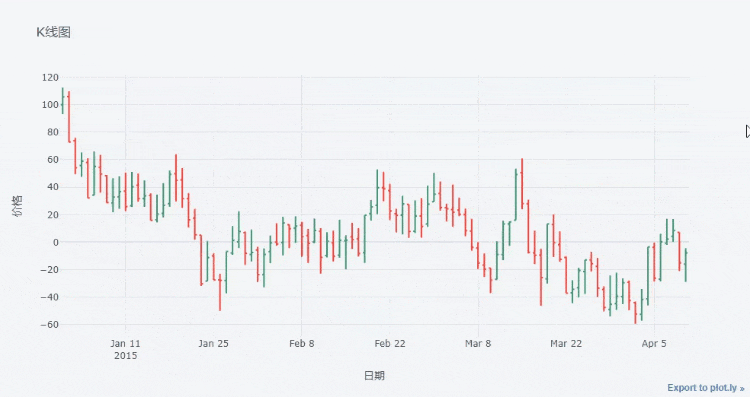### Histogram

``````df = pd.DataFrame({'a': np.random.randn(1000) + 1, 'b': np.random.randn(1000),
'c': np.random.randn(1000) - 1}, columns=['a', 'b', 'c'])
df.iplot(kind = "histogram")
Copy code ``````

output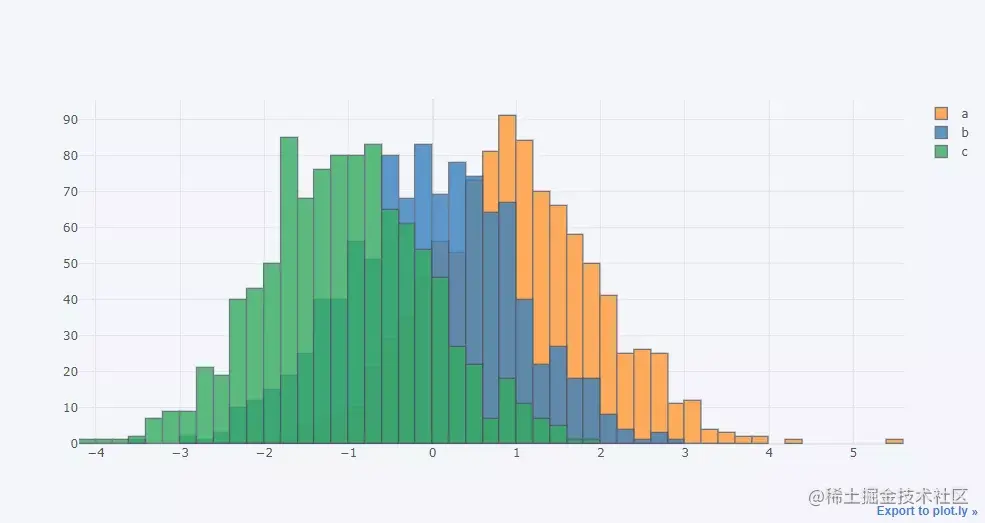### Drawing of multiple subgraphs

Then let's look at the drawing of multiple subgraphs , One is to use `scatter_matrix()` Method to implement

``````df = pd.DataFrame(np.random.randn(1000, 4),
columns=['a', 'b', 'c', 'd'])
df.scatter_matrix()
Copy code ``````

output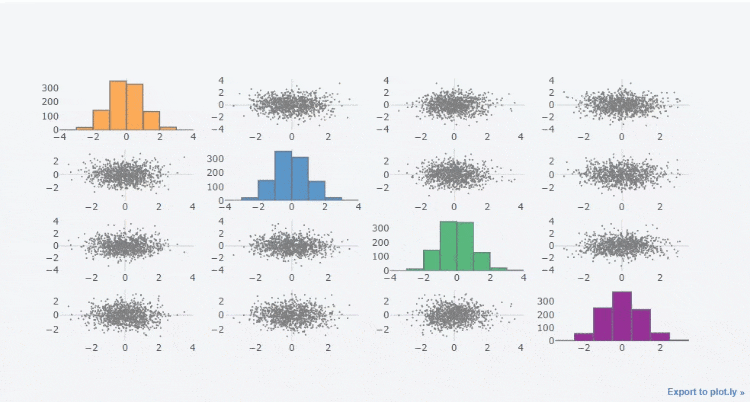The other is to use `subplots` Parameters , Set its parameters to `True`, For example, let's draw multiple histogram subgraphs

``````df_h=cf.datagen.histogram(4)
df_h.iplot(kind='histogram',subplots=True,bins=50)
Copy code ``````

output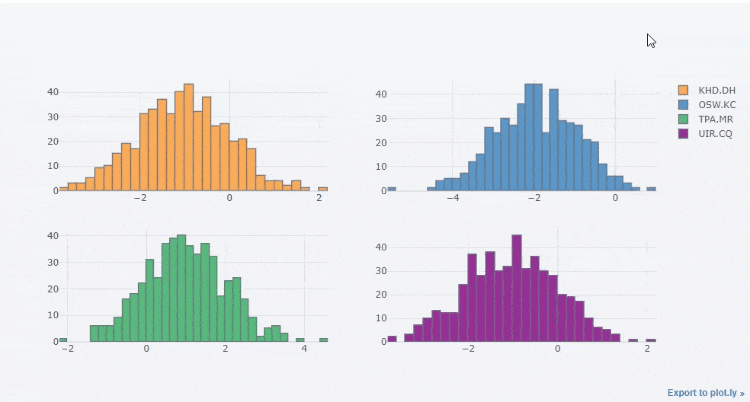Or draw multiple line graph subgraphs

``````df=cf.datagen.lines(4)
df.iplot(subplots=True,subplot_titles=True,legend=True)
Copy code ``````

output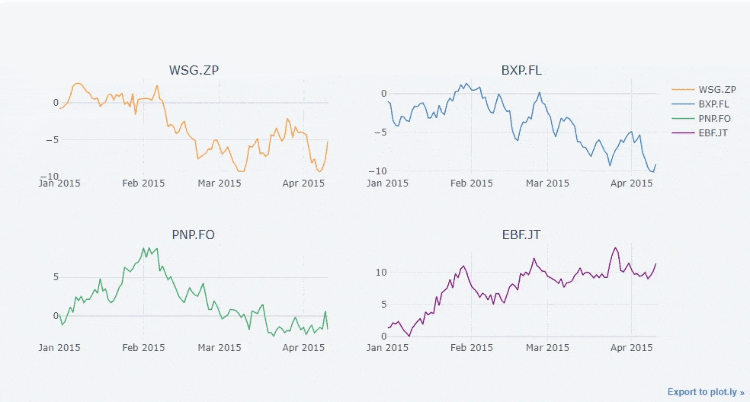Finally, we can freely combine the drawing of multiple subgraphs , Through the inside `specs` Parameters

``````df=cf.datagen.bubble(10,50,mode='stocks')
#  Define the form of the chart to be drawn
figs=cf.figures(df,[dict(kind='histogram',keys='x',color='blue'),
dict(kind='scatter',mode='markers',x='x',y='y',size=5),
dict(kind='scatter',mode='markers',x='x',y='y',size=5,color='teal')],asList=True)
figs.append(cf.datagen.lines(1).figure(bestfit=True,colors=['blue'],bestfit_colors=['red']))
base_layout=cf.tools.get_base_layout(figs)
#  How multiple subgraphs are distributed ,specs Among the parameters , It is divided into two rows and two columns for distribution
specs=cf.subplots(figs,shape=(3,2),base_layout=base_layout,vertical_spacing=.25,horizontal_spacing=.04,
specs=[[{'rowspan':2},{}],[None,{}],[{'colspan':2},None]],
subplot_titles=[' Histogram ',' Scatter plot _1',' Scatter plot _2',' Broken line diagram + Fitting line '])
specs['layout'].update(showlegend=True)
cf.iplot(specs)
Copy code ``````

output